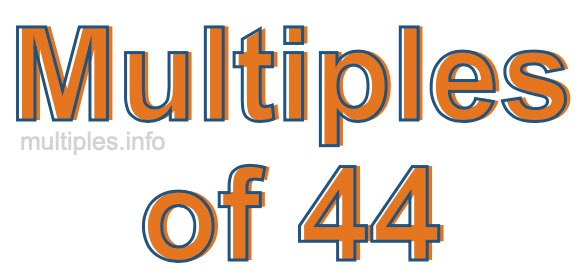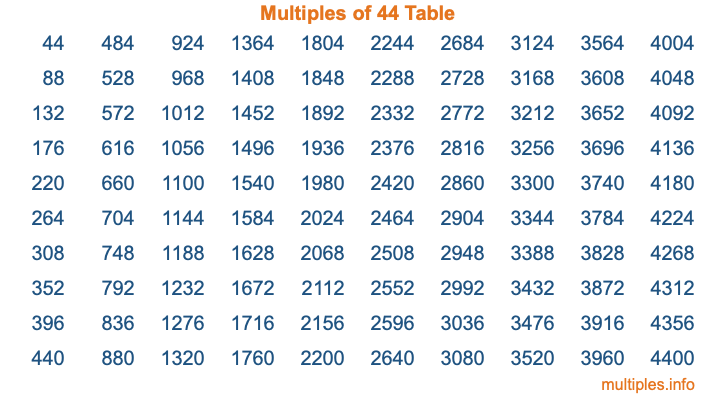Multiples of 44Welcome to the Multiples of 44 page. Here we will first teach you everything you will ever need to know about the multiples of 44, and then give you a study guide summary of everything we taught you to make sure you remember it all. Use this page to look up facts and learn information about the multiples of 44. This page will make you a multiples of forty-four expert!

Definition of Multiples of 44
Multiples of 44 are all the numbers that when divided by 44 equal an integer. Each of the multiples of 44 are called a multiple. A multiple of 44 is created by multiplying 44 by an integer.

Therefore, to create a list of multiples of 44, you start with 1 multiplied by 44, then 2 multiplied by 44, then 3 multiplied by 44, and so on for as long as you want. Thus, the list of the first five multiples of 44 is 44, 88, 132, 176, and 220. To see a larger list of multiples of 44, see the printable image of Multiples of 44 further down on this page. We also have a category where you can choose any nth multiple of 44.

Multiples of 44 Checker
The Multiples of 44 Checker below checks to see if any number of your choice is a multiple of 44. In other words, it checks to see if there is any number (integer) that when multiplied by 44 will equal your number. To do that, we divide your number by 44. If the the quotient is an integer, then your number is a multiple of 44.

Is  a multiple of 44?

Least Common Multiple of 44 and ...
A Least Common Multiple (LCM) is the lowest multiple that two or more numbers have in common. This is also called the smallest common multiple or lowest common multiple and is useful to know when you are adding our subtracting fractions. Enter one or more numbers below (44 is already entered) to find the LCM.

Check out our LCM Calculator if you need more details about the Least Common Multiple or if you need the LCM for different numbers for adding and subtraction fractions.

nth Multiple of 44
As we stated above, 44 is the first multiple of 44, 88 is the second multiple of 44, 132 is the third multiple of 44, and so on. Enter a number below to find the nth multiple of 44.

th multiple of 44

Multiples of 44 vs Factors of 44
44 is a multiple of 44 and a factor of 44, but that is where the similarities end. All postive multiples of 44 are 44 or greater than 44. All positive factors of 44 are 44 or less than 44.

Below is the beginning list of multiples of 44 and the factors of 44 so you can compare:

Multiples of 44: 44, 88, 132, 176, 220, etc.

Factors of 44: 1, 2, 4, 11, 22, 44

As you can see, the multiples of 44 are all the numbers that you can divide by 44 to get a whole number. The factors of 44, on the other hand, are all the whole numbers that you can multiply by another whole number to get 44.

It's also interesting to note that if a number (x) is a factor of 44, then 44 will also be a multiple of that number (x).

Multiples of 44 vs Divisors of 44
The divisors of 44 are all the integers that 44 can be divided by evenly. Below is a list of the divisors of 44.

Divisors of 44: 1, 2, 4, 11, 22, 44

The interesting thing to note here is that if you take any multiple of 44 and divide it by a divisor of 44, you will see that the quotient is an integer.

Multiples of 44 Table
Below is an image of the first 100 multiples of 44 in a table. The table is in chronological order, column by column. The first column has the first ten multiples of 44, the second column has the next ten multiples of 44, and so on.The Multiples of 44 Table is also referred to as the 44 Times Table or Times Table of 44. You are welcome to print out our table for your studies.

Negative Multiples of 44
Although not often discussed or needed in math, it is worth mentioning that you can make a list of negative multiples of 44 by multiplying 44 by -1, then by -2, then by -3, and so on, to get the following list of negative multiples of 44:

-44, -88, -132, -176, -220, etc.

Multiples of 44 Summary
Below is a summary of important Multiples of 44 facts that we have discussed on this page. To retain the knowledge on this page, we recommend that you read through the summary and explain to yourself or a study partner why they hold true.

There are an infinite number of multiples of 44.

A multiple of 44 divided by 44 will equal a whole number.

44 divided by a factor of 44 equals a divisor of 44.

The nth multiple of 44 is n times 44.

The largest factor of 44 is equal to the first positive multiple of 44.

44 is a multiple of every factor of 44.

44 is a multiple of 44.

A multiple of 44 divided by a divisor of 44 equals an integer.

44 divided by a divisor of 44 equals a factor of 44.

Any integer times 44 will equal a multiple of 44.

Multiples of a Number
Here you can get the multiples of another number, all with the same attention to detail as we did for multiples of 44 on this page.

Multiples of
Multiples of 45
Did you find our page about multiples of forty-four educational? Do you want more knowledge? Check out the multiples of the next number on our list!